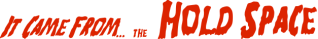Next42

# Rational arithmetic

• Multiplication is simple:

```        # Instead of  z = \$(( x * y )), use:
z_n = \$(( x_n * y_n ))
z_d = \$(( x_d * y_d ))
```
• So is division:

```        # Instead of  z = \$(( x / y )), use:
z_n = \$(( x_n * y_d ))
z_d = \$(( x_d * y_n ))
```
• Addition and subtraction are not much harder:

```        # Instead of  z = \$(( x - y )), use:
z_n = \$(( x_n * y_d - x_d * y_n ))
z_d = \$(( x_d * y_d ))
```